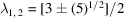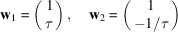International
Tables for
Crystallography
Volume B
Reciprocal space
Edited by U. Shmueli

International Tables for Crystallography (2010). Vol. B, ch. 4.6, pp. 597-598   | 1 | 2 |

## Section 4.6.2.5. 1D structures with fractal atomic surfaces

W. Steurera* and T. Haibacha

aLaboratory of Crystallography, Department of Materials, ETH Hönggerberg, HCI G 511, Wolfgang-Pauli-Strasse 10, CH-8093 Zurich, Switzerland
Correspondence e-mail:  walter.steurer@mat.ethz.ch

#### 4.6.2.5. 1D structures with fractal atomic surfaces

| top | pdf |

A 1D structure with a fractal atomic surface (Hausdorff dimension 0.9157…) can be derived from the Fibonacci sequence by squaring its substitution matrix S:corresponding to the substitution rule,as well as two other non-equivalent ones (see Janssen, 1995). The eigenvaluesare obtained by calculatingThe evaluation of the determinant gives the characteristic polynomialwith the solutions, withand, and the same eigenvectorsas for the Fibonacci sequence. Rewriting the eigenvalue equation givesIdentifying the eigenvectorwithshows that the infinite 1D sequencemultiplied by powers of its eigenvalue(scaling operation) remains invariant (each new lattice point coincides with one of the original lattice):The fractal sequence can be described on the same reciprocal and direct bases as the Fibonacci sequence. The only difference in the 2D direct-space description is the fractal character of the perpendicular-space component of the hyperatoms (Fig. 4.6.2.12)(see Zobetz, 1993).Figure 4.6.2.12 | top | pdf |(a) Three steps in the development of the fractal atomic surface of the squared Fibonacci sequence starting from an initiator and a generator. The action of the generator is to cut a piece from each side of the initiator and to add it where the initiator originally ended. This is repeated, cutting thinner and thinner pieces each time from the generated structures. (b) Magnification sequence of the fractal atomic surface illustrating its self-similarity. Each successive figure represents a magnification of a selected portion of the previous figure (from Zobetz, 1993).

### References

Janssen, T. (1995). From quasiperiodic to more complex systems. In Beyond Quasicrystals, edited by F. Axel & D. Gratias, pp. 75–140. Les Ulis: Les Editions de Physique and Berlin: Springer-Verlag.
Zobetz, E. (1993). One-dimensional quasilattices: fractally shaped atomic surfaces and homometry. Acta Cryst. A49, 667–676.